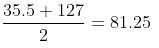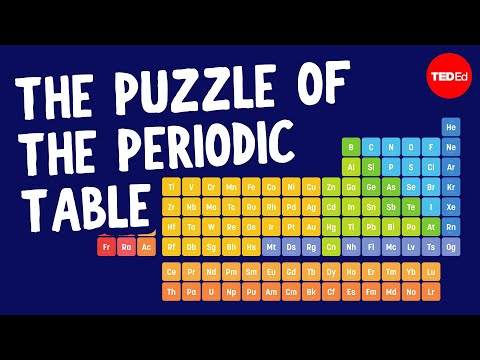Courses

# Test: Earlier Attempts of Classification of Elements

## 20 Questions MCQ Test Chemistry for JEE | Test: Earlier Attempts of Classification of Elements

Description
This mock test of Test: Earlier Attempts of Classification of Elements for JEE helps you for every JEE entrance exam. This contains 20 Multiple Choice Questions for JEE Test: Earlier Attempts of Classification of Elements (mcq) to study with solutions a complete question bank. The solved questions answers in this Test: Earlier Attempts of Classification of Elements quiz give you a good mix of easy questions and tough questions. JEE students definitely take this Test: Earlier Attempts of Classification of Elements exercise for a better result in the exam. You can find other Test: Earlier Attempts of Classification of Elements extra questions, long questions & short questions for JEE on EduRev as well by searching above.
QUESTION: 1

### Lothar Meyer arranged the elements in order of increasing:

Solution:

Lothar Meyer proposed that on arranging the elements in order of increasing atomic weights; similarities appear in physical and chemical properties.

QUESTION: 2

### Law of Octaves was proposed by:

Solution:

Law of octaves
The generalization made by the English chemist J.A.R. Newlands in 1865 that, if the chemical elements are arranged according to increasing atomic weight, those with similar physical and chemical properties occur after each interval of seven elements.

QUESTION: 3

### Law of Triads is applicable to which set of elements?

Solution:

For Dobereiner's triads, the atomic weight of a middle element is almost average of the other two. Thus, the law of triad is applicable to Cl,Br,IQUESTION: 4

In Mendeleev’s periodic table, the groups were divided into how many subgroups?

Solution:

Mendeleev divided his periodic table in eight groups and seven periods. Groups from I to VII are meant for normal elements and group VIII is for transition elements. Groups from I to VII have been divided in two sub groups, while group VIII is meant for three elements.

QUESTION: 5

Modern periodic table is divided into:

Solution:
• Elements are arranged on the basis of increasing order of atomic numbers.
• The horizontal rows in the periodic table are called Periods and the vertical columns are known as the Groups.
• The elements in the Modern Periodic Table are arranged in 7 Periods and 18 Groups.
• The elements in the Modern Periodic Table are classified into four categories viz. Representative Elements, Transition Elements, Inner Transition Elements, and Noble Gases.
QUESTION: 6

Moseley performed experiments and studied the frequencies of which radiations emitted from the elements?

Solution:

The origins of Moseley's conclusion that there was a whole number relationship in the frequencies of X-rays emitted from atoms after electron bombardment, i.e., the discovery of atomic numbers.

QUESTION: 7

Among the following statements the one that is not true about Mendeleev’s Periodic Table is:

Solution:

In Mendeleev’s periodic table, elements were arranged on the basis of atomic weights.

QUESTION: 8

Which scientist proposed that atomic number is more fundamental property of an element than its atomic mass?

Solution:

The scientist who first of all showed that the atomic number is more fundamental property of an element than its atomic mass was Henry Moseley.

QUESTION: 9

Johann Dobereiner classified elements in group of three elements called as:

Solution:

In 1829, Johann Dobereiner, a German scientist made some groups of three elements each and called them triads.

He observed that the atomic mass of the middle element of a triad was nearly equal to the arithmetic mean of the atomic masses of the other two elements. All three elements of a triad were similar in their properties.

QUESTION: 10

In the modern periodic table, which period contains 32 elements?

Solution:

The 2nd and 3rd period consists of 8 elements each. On the other hand, the 4th and 5th consists of 18 elements each while the sixth and seventh period consists of 32 elements.

QUESTION: 11

Horizontal rows in the periodic table are called:

Solution:

There are totally 7 horizontal rows are there they are called periods
Vertical columns are called groups or families.

QUESTION: 12

The second and third period of the modern periodic table contains how many elements in all?

Solution:

In second and third period of the modern periodic table does not involve any d orbital so we have s and p which have 8 electrons combined. So for the second period we have eight elements and for the third period we have 8 element so we have total 16 elements in all.

QUESTION: 13

Newland arranged elements in increasing order of atomic weights and noted that every eighth element had properties similar to:

Solution:

According to Newlands' law of octaves when the elements are arranged in order of increasing atomic weights then every eighth element has properties similar to that of the first element.

QUESTION: 14

According to Dobereiner’s law of triads the number of elements present in each group is:

Solution:

According to Dobereiner's law of triads each triad contains three elements. He also noticed that the middle element of each of the triad had an atomic weight about half way between atomic weights of the other two.

QUESTION: 15

Lothar Meyer proposed that on arranging the elements in order of increasing atomic weights; similarities appear in which type of properties?

Solution:

Lothar Meyer proposed that on arranging the elements in order of increasing atomic weights similarities appear in both physical and chemical properties.

QUESTION: 16

Eka silicon predicted by Mendeleev is which element:

Solution:

Mendeleev predicted the existence of 'eka-silicon', which would fit into a gap next to silicon. The element germanium was discovered later. Its properties were found to be similar to the predicted ones and confirmed Mendeleev's periodic table.

QUESTION: 17

Eka aluminium predicted by Mendeleev is which element?

Solution:

Eka aluminium predicted by Mendeleev is Gallium. Eka-aluminium and gallium are the two names of the same element as Eka -Aluminum has almost exactly the same properties as the actual properties of the gallium element.

QUESTION: 18

The basis of long form of periodic table is:

Solution:

In long form of periodic table elements are arranged in increasing order of their Atomic number.

QUESTION: 19

Mendeleev predicted the existence of which element/elements in the periodic table?

Solution:

Gallium and Germanium were the elements not discovered at that time and Mendeleev put gaps in the periodic table.
Gallium was called as Eka aluminium
Germanium was called as Eka silicon

QUESTION: 20

At present how many elements are known?

Solution:

There are 118 elements on the periodic table, Four with atomic numbers – 113 (Nihonium), 115 (Moskovi), 117 (Tennesin) and 118 (Oganesson) – were added in 2016. With the discoveries of new elements, it's difficult to ascertain how long the table is going to be in the future.

Track your progress, build streaks, highlight & save important lessons and more!

### Similar Content### Related tests